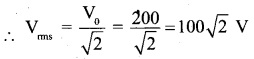# 2nd PUC Physics Question Bank Chapter 7 Alternating Current

## Karnataka 2nd PUC Physics Question Bank Chapter 7 Alternating Current

### 2nd PUC Physics Alternating Current NCERT Text Book Questions and Answers

Question 1.
A 100 Ω resistor is connected to a 220 V, 50 Hz ac supply.
(a) What is the rms value of current in the circuit?
(b) What is the net power consumed over a full cycle?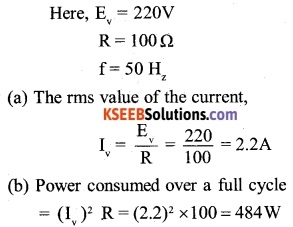Question 2.
(a) The peak voltage of an ac supply is 300 V. What is the rms voltage?
(b) The rms value of current in an ac circuit is 10 A. What is the peak current?Question 3.
A 44 mH inductor is connected to 220 V, 50 Hz ac supply. Determine the rms value of the current in the circuit.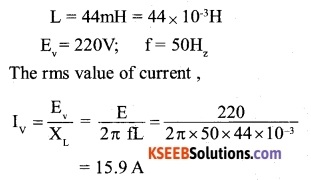Question 4.
A 60 µF capacitor is connected to a 110 V, 60 Hz ac supply. Determine the rms value of the current in the circuit.Question 5.
In Exercises 7.3 and 7.4, what is the net power absorbed by each circuit over a complete cycle. Explain your answer.
For Ex.7.3- The current lags behind the emf by a phase angle $$\frac { \pi }{ 2 }$$, when a.c. flows through an inductor.Therefore, power absorbed by inductor over a complete cycle is,Question 6.
Obtain the resonant frequency ωr of a series LCR circuit with L = 2.0H, C = 32 µ F and R = 10Ω . What is the Q-value of this circuit?Question 7.
A charged 30 pF capacitor is connected to a 27 mH inductor. What is the angular frequency of free oscillations of the circuit?Question 8.
Suppose the initial charge on the capacitor in Exercise 7.7 is 6 mC. What is the total energy stored in the circuit initially? What is the total energy at later time?
q=6mC = 6×10-3C
Initially, energy is stored in the capacitor and is given by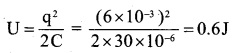The initial energy stored in the capacitor is shared by the inductor during oscillations of the circuit, but the total energy remains the same even at a later time.

Question 9.
A series LCR circuit with R = 20 Ω, L = 1.5 H and C = 35 µ F is connected to a variable-frequency 200 V ac supply. When the frequency of the supply equals the natural frequency of the circuit, what is the average power transferred to the circuit in one complete cycle?
When XL = Xc, Z = R
p = $$\frac{\mathrm{V}^{2}}{\mathrm{R}}$$
= $$\frac { 200×200 }{ 20 }$$
= 2KW

Question 10.
A radio can tune over the frequency range of a portion of MW broadcast band: (800 kHz to 1200 kHz). If its LC circuit has an effective inductance of 200 µH, what must be the range of its variable capacitor? [Hint: For tuning, the natural frequency i.e., the frequency of free oscillations of the LC circuit should be equal to the frequency of the radio wave.]
L = 200 p H = 200×10-6H
= 800 KHz to 1200 KHz
= 8×105Hz to 12 ×105Hz .
For tuning the radio, the natural frequency of the LC circuit should be equal to the frequency of the signal.
Now,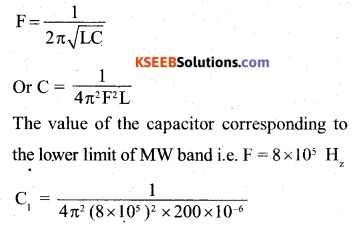Therefore, variable capacitor should be of range 88 pF to 198 pF.Question 11.
Figure 7.21 shows a series LCR circuit connected to a variable frequency 230 V source. L = 5.0 H, C = 80 µF, R – 40 Ω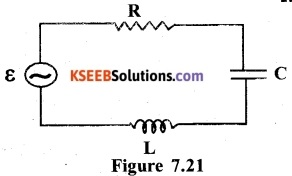(a) Determine the source frequency which drives the circuit in resonance.
(b) Obtain the impedance of the circuit and the amplitude of current at the resonating frequency.
(c) Determine the rms potential drops across the three elements of the circuit. Show that the potential drop across the LC combination is zero at the resonating frequency.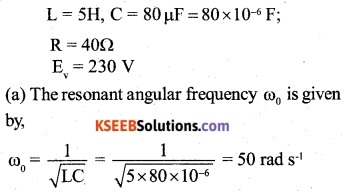(b) At resonance, the impedance of the LCR circuit is simply equal to R
∴ at resonance, Z = R = 40 Ω
The rms value of current at resonance frequency is,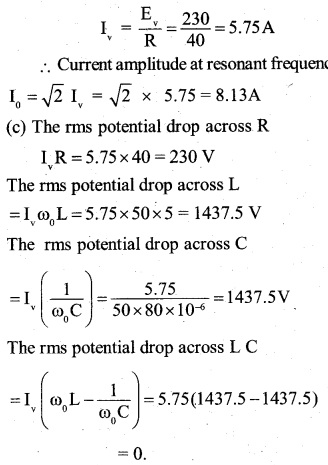### 2nd PUC Physics Alternating Current Additional Exercises

Question 12.
An LC circuit contains a 20 mH inductor and a 50 µF capacitor with an initial charge of 10 mC. The resistance of the circuit is negligible. Let the instant the circuit is closed be t = 0.
(a) What is the total energy stored initially? Is it conserved during LC oscillations?
(b) What is the natural frequency of the circuit?
(c) At what time is the energy stored
(d) completely electrical (i.e., stored in the capacitor)?
(e) completely magnetic (i.e., stored in the inductor)?
(f) At what times is the total energy shared equally between the inductor and the capacitor?
(e) If a resistor is inserted in the circuit, how much energy is eventually dissipated as heat?
L = 20mH = 20×103 H
C = 50 µF = 50 x 10-6 F
charge on the capacitor initially,(d) The total energy is shared equally between the inductor and the capacitor, when the energy stored in capacitor becomes equal to one half of the total energy. If q is charge on the capacitor at that instant, then(e) When resistance is introduced, the whole of the energy will be dissipated in the form of heat. The introduction of resistance produces damped oscillations.Question 13.
A coil of inductance 0.50 H and resistance 100 Q is connected to a 240 V, 50 Hz ac supply.
(a) What is the maximum current in the coil?
(b) What is the time lag between the voltage maximum and the current maximum?Question 14.
Obtain the answers (a) to (b) in Exercise 7.13 if the circuit is connected to a high-frequency supply (240 V, 10 kHz). Hence, explain the statement that at very high frequency, an inductor in a circuit nearly amounts to an open circuit. How does an inductor behave in a dc circuit after the steady-state?In Ex-7.13, H was obtained that, when F = 50H, I = 1.289 A However, when frequency is very high (≈10kHz), the current drops to 1.08 x 10-2A (nearly zero). Hence at very high frequency in an a.c. circuit, an inductor nearly amounts to an open circuit.
For d.c. supply, F = 0
∴ Y = 2πFL = 2π x 0 x 0.50 = 0
Thus in a d.c. circuit, inductor offers no resistance i.e. it behaves like a conductor.

Question 15.
A 100 pF capacitor in series with a 40 fit resistance is connected to a 110 V, 60 Hz supply.
(a) What is the maximum current in the circuit?
(b) What is the time lag between the current maximum and the voltage maximum?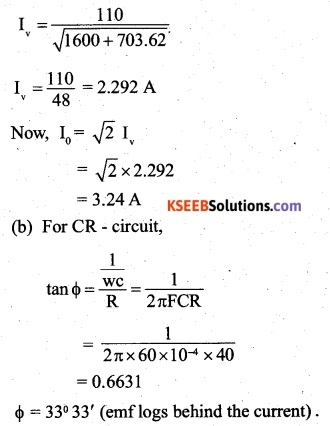Question 16.
Obtain the answers to (a) and (b) in Exercise 7.15 if the circuit is connected to a 110 V, 12 kHz supply? Hence, explain. the statement that a capacitor is a conductor at very high frequencies. Compare this behaviour with that of a capacitor in a dc circuit after the steady-state.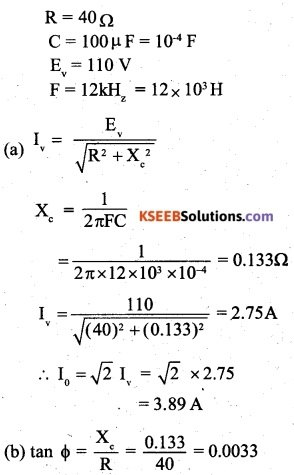Or φ = 0.2° ≈ 0° ;
In absence of capacitor, current through the circuit
$$=\frac { { E }_{ v } }{ R } =\frac { 110 }{ 40 } =2.75A$$
It follows that when frequency is very high (≈ 12kHz) current in the circuit is same, whether capacitor is connected in the circuit or not. In other words, at very high frequency, the capacitor behaves like a conductor in an a.c. circuit.
For d.c. supply,f=0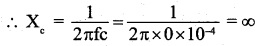Thus in d.c, circuit, a capacitor amounts to an open circuit (offer infinite resistance).

Question 17.
Keeping the source frequency equal to the resonating frequency of the series LCR circuit, if the three elements, L, C and R are arranged in parallel, show that the total current in the parallel LCR circuit is minimum at this frequency. Obtain the’ current rms value in each branch of the circuit for the elements and source specified in Exercise 7.11 for this frequency.
From the phasor diagram, it can be obtained that when the three elements L, C and R are arranged in parallel, the impedance of the circuit is given by,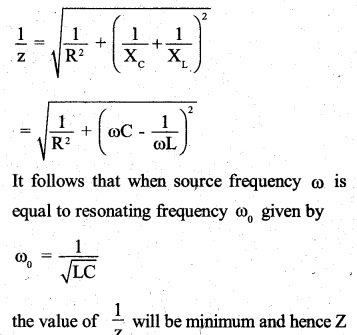will be maximum and equal to R. Likewise, the current amplitude will be minimum.
L = 5H;C = 80 pF = 80 x 10-6F; R = 40q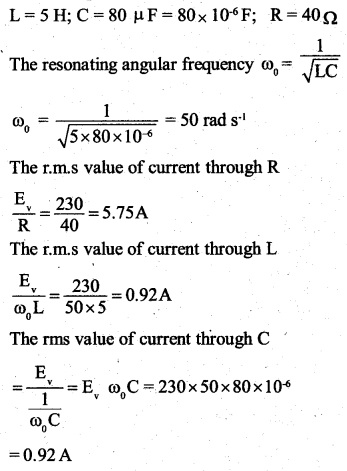Question 18.
A circuit containing an 80 mH inductor and a 60 µF capacitor in series is connected to a 230 V, 50 Hz supply. The resistance . of the circuit is negligible.
(a) Obtain the current amplitude and ran values.
(b) Obtain the rms values of potential drops across each element.
(c) What is the average power transferred to the inductor?
(d) What is the average power transferred to the capacitor?
(e) What is the total average power absorbed by the circuit?
[‘Average’ implies ‘averaged over one cycle’.]
L = 80mH = 80×10-3H
C = 60pF = 60 x 10-6F
E = 230 V
F = 50 HzQuestion 19.
Suppose the circuit in Exercise 7.18 has a resistance of 15 Ω Obtain the average power transferred to each element of the circuit, and the total power absorbed.
Ans:
R = 15Ω
L = 80 mH = 80 x 10-3 H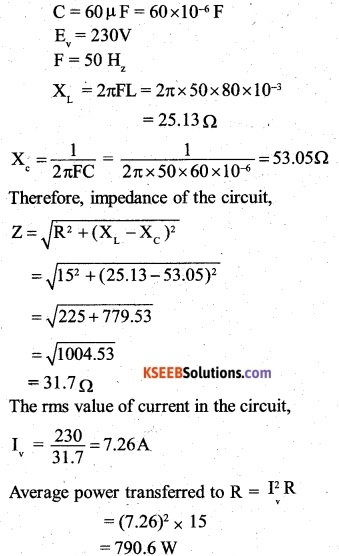Average power transferred to both L and C is zero. It can be checked that the average power of the circuit is equal to the average power transferred to R.Question 20.
A series LCR circuit with L = 0.12 H, C = 480 nF, R = 23 O is connected to a 230 V variable frequency supply.
(a) What is the source frequency for which current amplitude is maximum. Obtain this maximum value.
(b) What is the source frequency for which average power absorbed by the circuit is maximum. Obtain the value of this maximum power.
(c) For which frequencies of the source are the power transferred to the circuit half the power at resonant frequency? What is the current amplitude at these frequencies?
(d) What is the Q-factor of the given circuit?
L = 0.12H
C = 480nF = 480 x 10-9F
R = 23 Ω
Ev= 230 V
(a) Current amplitude is maximum at resonant frequency.
The resonant frequency is given by 1(b) Average power will also be maximum at resonant frequency i.e. at 4166.7 rad s-1 P =E I cos Φ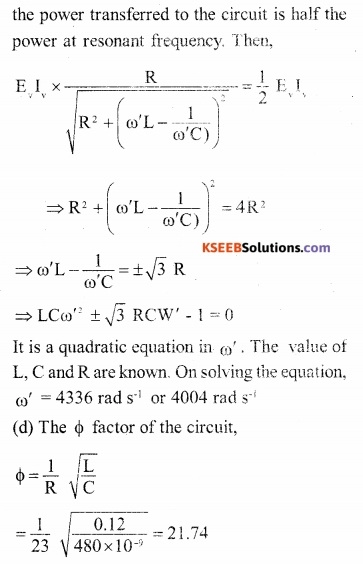Question 21.
Obtain the resonant frequency and Q-factor of a series LCR circuit with L = 3.0 H, C = 27 (an F, and R = 7.4 Ω It is desired to improve the sharpness of the resonance of the circuit by reducing its ‘full, width at half maximum’ by a factor of 2. Suggest a suitable way.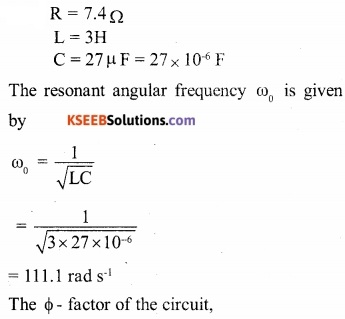The simplest way to improve the sharpness of the resonance of the circuit is by reducing its ‘ full width at half maximum by a factor of 2 is to reduce the value of R to $$\frac { R }{ 2 }$$ i.e. by making
$$R=\frac { 7.4 }{ 2 } =3.7\Omega$$

Question 22.
(a) In any ac circuit, is the applied instantaneous voltage equal to the algebraic sum of the instantaneous voltages across the series elements of the circuit? Is the same true for rms voltage?

(b) A capacitor is used in the primary circuit of an induction coil.                               ‘

(c) An applied voltage signal consists of a superposition of a dc voltage and an ac voltage of high frequency. The circuit consists of an inductor and a capacitor in series. Show that the dc signal will appear across C and the ac signal across

(d) A choke coil in series with a lamp is connected to a dc line. The lamp is seen to shine brightly. Insertion of an iron core in the choke causes no change in the lamp’s brightness. Predict the corresponding observations if the connection is to an ac line.

(e) Why is choke coil needed in the use of fluorescent tubes with ac mains? Why can we not use an ordinary resistor instead of the choke coil?
Ans:
(a) Yes, the first part is true but it is incorrect for r.m.s. voltage because the potential difference across various components may not be in phase.

(b) When the primary circuit of the induction coil breaks, high voltage is induced which is used to charge the capacitor. This avoids sparking in the circuit.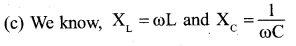XL has zero value for d.c and a high value for a.c while Xc has an infinite value for d.c and a low value for a.c. When the voltage signal consisting of a superposition of .d.c voltage and an a.c voltage is applied to the series combination of a capacitor and an inductor, the inductor will oppose a.c, whereas it will allow easy path to d.c. Therefore, a.c voltage will appear across L. For a similar reason, d.c. the voltage will appear across C.

(d) For d.c, XL = 0. It means, insertion of an iron core in choke or inductor does not affect XL. However, in case of a.c. XL = ωL = 2ΠvL. When iron core in a choke is inserted, value of L increases. Hence, current decreases and the brightness of the lamp decreases.

For d.c, XL = 0. It means, insertion of an iron core in choke or inductor does not affect XL. However, in case of a.c. XL = coL = 2n When iron core in a choke is inserted, value of L increases. Hence, current decreases and the brightness of the lamp decreases.

(e) Choke is an inductor which reduces the supply voltage to an appropriate value to operate the tube without any power loss. However, when the resistor is used, power is dissipated in the form of heat.

Question 23.
A power transmission line feeds input power at 2300 V to a step-down transformer with its primary windings having 4000 turns. What should be the number of turns in the secondary in order to get output power at 230 V?Question 24.
At a hydroelectric power plant, the water pressure head is at a height of 300 m and the water flow available is 100 mV. If the turbine generator efficiency is 60%, estimate the electric power available from the plant (g = 9.8 ms-2).Question 25.
A small town with a demand of 800 kW of electric power at 220 V is situated 15 km away from an electric plant generating power at 440 V. The resistance of the two wirelines carrying power is 0.5 Ω per km. The town gets power from the line through a 4000-220 V step-down transformer at a sub-station in the town.
(a) Estimate the line power loss in the form of heat.
(b) How much power must the plant supply, assuming there is negligible power loss due to leakage?
(c) Characterise the step-up transformer at the plant.
Length of the wire line = 15 x 2 = 30 km
Resistance of the wire line, R = 30 x 0.5 = 15Ω
The voltage at which power is transmitted – 4000 V
Power transmitted, P 800 kW = 8 x 105 W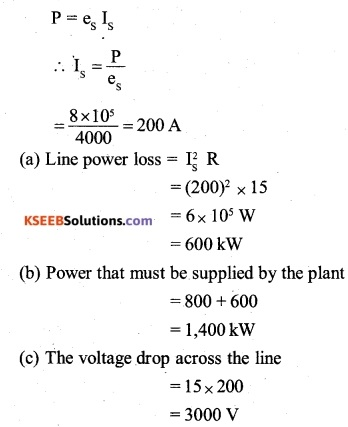The plant generates power at 440 V and it has to be stepped up so that after a voltage drop of 3000 V across the wireline, the power at 4000 V is received at the substation in the town. Therefore, the step-up transformer at the plant ranges from 440 V to (4000 + 3000) V i.e.440 V – 7000 V.Question 26
Do the same exercise as above with the replacement of the earlier transformer by a 40,000-220 V step-down transformer (Neglect, as before, leakage losses though this may not be a good assumption any longer because of the very high voltage transmission involved). Hence, explain why high voltage transmission is preferred?
Length of the wire line = 15 ×2 = 30Km.
Resistance of the wire line, R = 30 x 0.5=15q
Voltage at which power is transmitted = 40000 V
Power transmitted, P 800KW = 8 x 105 W(b) Power that must be supplied by plant = 800 + 6 = 806 kW

(c) Voltage drop across the wireline = 15 x 20 = 3000 V
Therefore, percentage power loss
$$=\frac { 6 }{ 806 } \times 100=42.86%$$
Since the power loss at high voltage transmission is negligible as compared to that at low voltage, the high voltage transmission of power is preferred.

Question 1.
Define root mean square value of an alternating current.  (CBSE 2010)
It is that steady current, which when passed through resistance for a given time will produce the same amount of heat as the alternating current does in the same resistance and at the same time.

Question 2.
Why d.c. voltmeter and d.c. the ammeter cannot read a.c?
It is because the average value of a.c. is zero over a complete cycle.

Question 3.
The frequency of a.c. the source is doubled. How do R, XL, and Xc get affected?
There is no effect of doubling the frequency of the a.c. source on R. Since XL = 2ΠfL, the value of XL becomes double on doubling the frequency of the a.c. source.
Further $${ X }_{ c }=\frac { 1 }{ 2\pi fc }$$
∴The value of Xc becomes half on doubling the frequency of the a.c. source.

Question 4.
In an oscillating LC- circuit, the maximum charge on the capacitor is Q. The charge on the capacitor, when the energy is stored equally between the electric and magnetic field is
(A) $$\frac { Q }{ 2 }$$
(B) $$\frac { Q }{ \sqrt { 3 } }$$
(C) $$\frac { Q }{ \sqrt { 2 } }$$
(D) Q
(C) $$\frac { Q }{ \sqrt { 2 } }$$
Let C be the capacitance of the capacitor. When the charge on the capacitor is Q, the energy stored inside it is given by
$${ U }_{ C }\frac { { Q }^{ 2 } }{ 2 }$$
Let Q1 be a charge on the capacitor when the energy is stored equally between the electric and magnetic fields. Then,
$${ U }_{ c }\frac { { U }_{ c } }{ 2 }$$Question 5.
In a circuit, L, C, and R are connected in series with an alternating voltage source of frequency f. The current leads the voltage by 45°. The value of C isφ = 45°
In LCR circuit, the phase difference between current and emf is given byQuestion 6.
The reactance of a capacitor of capacitance C is X. If both the frequency and capacitance be doubled, then new reactance will be
(A) X
(B) 2X
(C) 4X
(D) $$\frac { X }{ 4 }$$
Xc = X
$$\frac { 1 }{ 2\pi fC } =X$$
When both capacitance and frequency are doubled, the reactance will become,Question 7.
The coil of a choke in a circuit
(A) Increases the current
(B) Decreases the current
(C) Does not change the current
(D) Has high resistance to d.c circuit
(B) Decreases the current

Question 8.
A normal domestic electric supply is an alternating current, whose average value is
(A) zero
(B) half the peak value
(C) the peak value multiplied by $$\left( \frac { \pi }{ 2 } \right)$$
(D) The peak value divided by $$\left( \frac { \pi }{ 2 } \right)$$
(D) The peak value divided by $$\left( \frac { \pi }{ 2 } \right)$$

Question 9.
The equation of an a.c. voltage is V = 200 sin 50 mt . Then, the rms value of voltage is
(A) $$100\sqrt { 2 } V$$
(B) $$200\sqrt { 2 } V$$
(C) 100 V
(D) 400 V